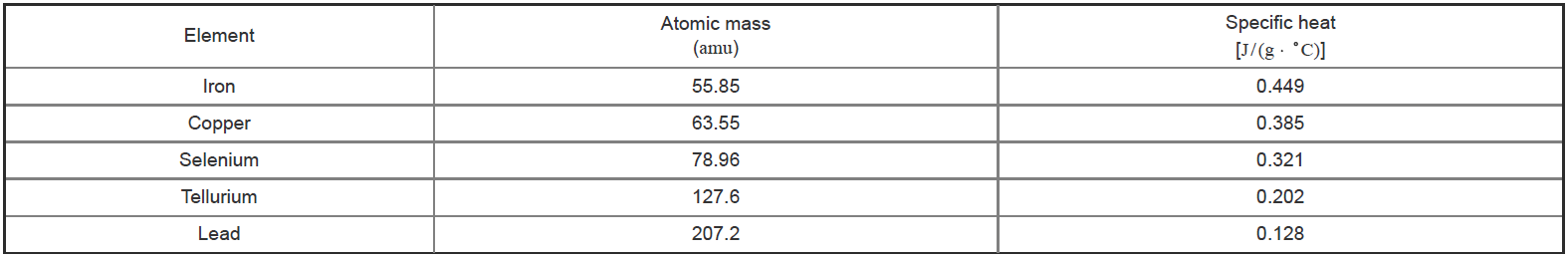# Problem: Raising the temperature of 32.0 g of a particular metal by 25 °C required 456 J of heat. What is the most likely identity of this metal?Enter the atomic symbol or element name. Equation: y = 25x + 0.015, where y represents specific heat and x represents the inverse of atomic massBased on specific heat measurements, Pierre Dulong and Alexis Petit proposed in 1818 that the specific heat of an element is related to its atomic weight (atomic mass). Thus, by measuring the specific heat of a new element, its atomic weight could be readily established. The specific heat and atomic mass for a series of elements are provided for reference.

###### FREE Expert Solution
98% (410 ratings)View Complete Written Solution
###### Problem Details

Raising the temperature of 32.0 g of a particular metal by 25 °C required 456 J of heat. What is the most likely identity of this metal?

Enter the atomic symbol or element name. Equation: y = 25x + 0.015, where y represents specific heat and x represents the inverse of atomic mass

Based on specific heat measurements, Pierre Dulong and Alexis Petit proposed in 1818 that the specific heat of an element is related to its atomic weight (atomic mass). Thus, by measuring the specific heat of a new element, its atomic weight could be readily established. The specific heat and atomic mass for a series of elements are provided for reference.